NEET  >  AIIMS Physics Mock Test - 5

# AIIMS Physics Mock Test - 5

Test Description

## 60 Questions MCQ Test AIIMS Mock Tests & Previous Year Papers | AIIMS Physics Mock Test - 5

AIIMS Physics Mock Test - 5 for NEET 2022 is part of AIIMS Mock Tests & Previous Year Papers preparation. The AIIMS Physics Mock Test - 5 questions and answers have been prepared according to the NEET exam syllabus.The AIIMS Physics Mock Test - 5 MCQs are made for NEET 2022 Exam. Find important definitions, questions, notes, meanings, examples, exercises, MCQs and online tests for AIIMS Physics Mock Test - 5 below.
Solutions of AIIMS Physics Mock Test - 5 questions in English are available as part of our AIIMS Mock Tests & Previous Year Papers for NEET & AIIMS Physics Mock Test - 5 solutions in Hindi for AIIMS Mock Tests & Previous Year Papers course. Download more important topics, notes, lectures and mock test series for NEET Exam by signing up for free. Attempt AIIMS Physics Mock Test - 5 | 60 questions in 60 minutes | Mock test for NEET preparation | Free important questions MCQ to study AIIMS Mock Tests & Previous Year Papers for NEET Exam | Download free PDF with solutions
 1 Crore+ students have signed up on EduRev. Have you?
AIIMS Physics Mock Test - 5 - Question 1

### In an AC circuit with voltage V and current I, the power dissipated is

AIIMS Physics Mock Test - 5 - Question 2

### A particle carrying a charge 'e' is fired perpendicularly in to a uniform magnetic field of induction B with a momentum 'p'; then the radius of circular path is

AIIMS Physics Mock Test - 5 - Question 3

### The space between the plates of a parallel plate capacitor is filled consecutively with two dielectric layers of thickness d1 and d2 having relative permittivities ε1 and ε2 respectively. If A is area of each plate, the capacity of the capacitor is

Detailed Solution for AIIMS Physics Mock Test - 5 - Question 3

As it is series combination of two capacitors,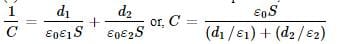AIIMS Physics Mock Test - 5 - Question 4

The rest mass of a photon is

AIIMS Physics Mock Test - 5 - Question 5

A sphere of mass m moving with a constant velocity u hits another stationary sphere of the same mass. If e is the coefficient of restitution, then the ratio of the velocity of two spheres after collision will be

AIIMS Physics Mock Test - 5 - Question 6

A wire of resistance 10Ω is elongated by 10%. The resistance of the elongated wire is

Detailed Solution for AIIMS Physics Mock Test - 5 - Question 6

the volume of the wire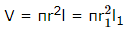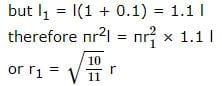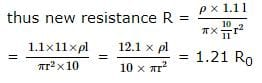Thus the elongated resistance is 1.21 times the resistance of original wire i.e 12.1

AIIMS Physics Mock Test - 5 - Question 7

When light of wavelength 300 nm (nanometer) falls on a photoelectric emitter, photoelectrons are liberated. For another emitter, however light of 600 nm wavelength is sufficient for creating photoemission. What is the ratio of the work functions of the two emitters

AIIMS Physics Mock Test - 5 - Question 8

A metal plate gets heated, when cathode rays strike against, it due to

AIIMS Physics Mock Test - 5 - Question 9

Which light when falling on a metal will emit photo electrons

AIIMS Physics Mock Test - 5 - Question 10

The coil of a dynamo is rotating in a magnetic field. The developed induced e.m.f. changes and the number of magnetic lines of force also changes. The phenomenon can be correctly described as

AIIMS Physics Mock Test - 5 - Question 11

The current flowing in a coil of self inductance 0.4 mH is increased by 250 mA in 0.1 s. The e.m.f. induced will be

Detailed Solution for AIIMS Physics Mock Test - 5 - Question 11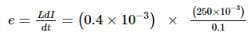= 10-3 V = 1mV
As current is increasing, induced e.m.f. is in opposite direction, therefore, e = - 1 mV.

AIIMS Physics Mock Test - 5 - Question 12

Determine the potential difference Va − Vb between the points a and b of the system of capacitors shown in figure. Given that the capacitance ratio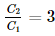and emf of the cell is E = 95 V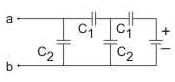AIIMS Physics Mock Test - 5 - Question 13

Dimensions of 1/μ₀ ∈₀, where symbols have their usual meanings, are

AIIMS Physics Mock Test - 5 - Question 14

A light bulb and a parallel plate capacitor (including a dielectric material between the plates) are connected in series to the 60-Hz ac voltage at a wall outlet. When the dielectric material is removed from the space between the plates, does the brightness of the bulb,

AIIMS Physics Mock Test - 5 - Question 15

In an L-R circuit, the value of L is (0.4/π) henry and the value of R is 30 ohm. If in the circuit, an alternating emf of 200 volt at 50 cycles per second is connected, the impedance of the circuit and current will be

Detailed Solution for AIIMS Physics Mock Test - 5 - Question 15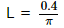henry, R = 30 ohm
e = 200 volt, f = 50
I = ?, Z = ?
Impedance of L-R circuit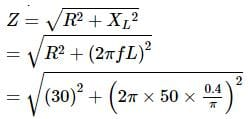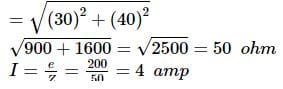AIIMS Physics Mock Test - 5 - Question 16

Inside a hollow charged spherical conductor, the potential at every point

AIIMS Physics Mock Test - 5 - Question 17

The same retarding force is applied to stop a train. The train stops after 80 m. If the speed is doubled, then the distance will be

AIIMS Physics Mock Test - 5 - Question 18

Two bodies of mass m₁ and m₂ have equal kinetic energies. If p₁ and p₂ are their respective momentum, then ratio p₁ : p₂ is equal to

AIIMS Physics Mock Test - 5 - Question 19

A black body has a wavelength of λ at temperature 2000 K. Its corresponding wavelength at temperature 3000 K will be

AIIMS Physics Mock Test - 5 - Question 20

Two bulbs of 500 W and 200 W are manufactured to operate on 220 V line. The ratio of heat produced in 500 W and 200 W, in two cases, when firstly they are connected in parallel and secondary in series will be

AIIMS Physics Mock Test - 5 - Question 21

The magnetic induction and the intensity of magnetic field inside an iron core of an electromagnet are 1 wb m-2 and 150 Am-1 respectively. The relative permeability of iron is (μ0 = 4π x 10−7 Henry ∕ m)

Detailed Solution for AIIMS Physics Mock Test - 5 - Question 21

We know that B = μH = μ0μrH
Where μ0 = permeability of free space or air
μr = relative permeability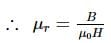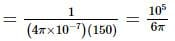AIIMS Physics Mock Test - 5 - Question 22

At the magnetic north pole of the earth, the value of the horizontal component of earth's magnetic field and angle of dip are respectively

AIIMS Physics Mock Test - 5 - Question 23

The Young's modulus of a wire of length (L) and radius (r) is Y N-m⁻2. If the length is reduced to L/2 and radius to r/2, then Young's modulus for the wire will be

AIIMS Physics Mock Test - 5 - Question 24

The motion of a rocket is based on the principle of conservation of

AIIMS Physics Mock Test - 5 - Question 25

The basis for carbon-14 dating method is that the

AIIMS Physics Mock Test - 5 - Question 26

The time period of a simple pendulum is 2 seconds. If length is increased by 4 times, then its period becomes

AIIMS Physics Mock Test - 5 - Question 27

In the following question, a Statement of Assertion (A) is given followed by a corresponding Reason (R) just below it. Read the Statements carefully and mark the correct answer-
Assertion(A): If velocity-time graph is a straight line parallel to time axis, then acceleration of body is zero.
Reason(R): Acceleration is equal to rate of change of velocity (constant).

AIIMS Physics Mock Test - 5 - Question 28

In the following question, a Statement of Assertion (A) is given followed by a corresponding Reason (R) just below it. Read the Statements carefully and mark the correct answer-
Assertion(A): When net force is perpendicular to direction of velocity, it just changes its direction and not magnitude.
Reason (R): This results in uniform circular motion.

AIIMS Physics Mock Test - 5 - Question 29

In the following question, a Statement of Assertion (A) is given followed by a corresponding Reason (R) just below it. Read the Statements carefully and mark the correct answer-
Assertion(A): A uniform sphere is placed on a smooth horizontal surface and a horizontal force F is applied on it at a distance h above the surface. The acceleration of centre is independent of h.
Reason(R): Acceleration depends only on force and mass.

AIIMS Physics Mock Test - 5 - Question 30

In the following question, a Statement of Assertion (A) is given followed by a corresponding Reason (R) just below it. Read the Statements carefully and mark the correct answer-
Assertion(A):γ-rays are not deflected by electronic and magnetic fields.
Reason(R):γ-rays have no change.

AIIMS Physics Mock Test - 5 - Question 31

In the following question, a Statement of Assertion (A) is given followed by a corresponding Reason (R) just below it. Read the Statements carefully and mark the correct answer-
Assertion(A):An astronaut accidentally gets separated out of his small spaceship accelerating in intersteller space at a constant rate of 90ms⁻2. The acceleration of the astronaut at the instant he is outside the spaceship is more than 90ms⁻2.
Reason(R):More forces act on the astronaut the moment he is out of spaceship.

AIIMS Physics Mock Test - 5 - Question 32

In the following question, a Statement of Assertion (A) is given followed by a corresponding Reason (R) just below it. Read the Statements carefully and mark the correct answer-
Assertion(A): Moving charge particle traces a helical path in a uniform magnetic field provided axis of the helix is perpendicular to magnetic field.
Reason(R): If a moving charges particle enters perpendicularly into a region of magnetic field from outside, it does not complete circular path.

AIIMS Physics Mock Test - 5 - Question 33

In the following question, a Statement of Assertion (A) is given followed by a corresponding Reason (R) just below it. Read the Statements carefully and mark the correct answer-
Assertion(A): Dimensions of Planck's constant are energy x time.
Reason(R): Energy = charge on electron x potential difference.

AIIMS Physics Mock Test - 5 - Question 34

In the following question, a Statement of Assertion (A) is given followed by a corresponding Reason (R) just below it. Read the Statements carefully and mark the correct answer-
Assertion(A):A bird is sitting on the floor of a wire cage and the cage is in the hand of a boy. Even when the bird starts flying in the cage, the boy does not experience any change in the weight of the cage.
Reason(R):Bird is still in the cage because of which the boy does not experience any change.

AIIMS Physics Mock Test - 5 - Question 35

In the following question, a Statement of Assertion (A) is given followed by a corresponding Reason (R) just below it. Read the Statements carefully and mark the correct answer-
Assertion(A): The ratio of the first three harmonics in a closed pipe bear a ratio 1:3:5.
Reason(R): Frequency is an odd multiple of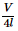where l is the length of the pipe.

AIIMS Physics Mock Test - 5 - Question 36

In the following question, a Statement of Assertion (A) is given followed by a corresponding Reason (R) just below it. Read the Statements carefully and mark the correct answer-
Assertion(A): Surface area of a drop of liquid reduces to reduce energy.
Reason(R): Molecules on the surface of a drop have more energy than the molecules in the interior.

AIIMS Physics Mock Test - 5 - Question 37

In the following question, a Statement of Assertion (A) is given followed by a corresponding Reason (R) just below it. Read the Statements carefully and mark the correct answer-
Assertion(A): Heavy stable nuclides tend to have more number of neutrons than protons.
Reason(R) : In heavy nuclei, there is an excess of neutrons due to Coulomb repulsion between protons.

AIIMS Physics Mock Test - 5 - Question 38

In the following question, a Statement of Assertion (A) is given followed by a corresponding Reason (R) just below it. Read the Statements carefully and mark the correct answer-
Assertion(A): Friction helps motion of body.
Reason(R): Friction is electromagnetic in motion.

AIIMS Physics Mock Test - 5 - Question 39

In the following question, a Statement-1 is given followed by a corresponding Statement-2 just below it. Read the statements carefully and mark the correct answer-
Statement-1:
In a Meter Bride experiment, null point for an unknown resistance is measured. Now, the unknown resistance is put inside an enclosure maintained at a higher temperature. The null point can be obtained at the same point as before by decreasing the value of the standard resistance.
Statement-2:
Resistance of a metal increases with increase in temperature.

AIIMS Physics Mock Test - 5 - Question 40

In the following question, a Statement of Assertion (A) is given followed by a corresponding Reason (R) just below it. Read the Statements carefully and mark the correct answer-
Assertion(A): Ionisation energy of atomic hydrogen is greater than atomic deuterium.
Reason(R): Ionisation energy is directly proportional to reduced mass.

AIIMS Physics Mock Test - 5 - Question 41

In the following question, a Statement of Assertion (A) is given followed by a corresponding Reason (R) just below it. Read the Statements carefully and mark the correct answer-
Assertion(A1): Frictional forces are conservative.
Reason(R): Potential energy can be associated with frictional forces.

AIIMS Physics Mock Test - 5 - Question 42

In the following question, a Statement of Assertion (A) is given followed by a corresponding Reason (R) just below it. Read the Statements carefully and mark the correct answer-
Assertion(A): We can distinguish a friend's voice, while listening music.
Reason(R) : Principle of superposition is a linear one.

AIIMS Physics Mock Test - 5 - Question 43

In the following question, a Statement of Assertion (A) is given followed by a corresponding Reason (R) just below it. Read the Statements carefully and mark the correct answer-
Assertion(A): Energy level of an atom with an vacancy in K-shell is below the L,M and N-shells that is lowest.
Reason(R): Electrons in K-shell are most tightly bound.

AIIMS Physics Mock Test - 5 - Question 44

In the following question, a Statement of Assertion (A) is given followed by a corresponding Reason (R) just below it. Read the Statements carefully and mark the correct answer-
Assertion(A): As the radius of earth reduces by 50% without any change in mass, length of a day reduces.
Reason(R): Angular momentum conservation provides drop in time as l decreases to 25% of the original.

AIIMS Physics Mock Test - 5 - Question 45

In the following question, a Statement-1 is given followed by a corresponding Statement-2 just below it. Read the statements carefully and mark the correct answer-
Statement-1:
For an observer looking out through the window of a fast moving train, the nearby objects appear to move in the opposite direction to the train, while the distant objects appear to be stationary.
Statement-2:
If the observer and the object are moving at velocities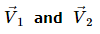respectively with reference to a laboratory frame, the velocity of the object with respect to the observer is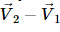AIIMS Physics Mock Test - 5 - Question 46

In the following question, a Statement of Assertion (A) is given followed by a corresponding Reason (R) just below it. Read the Statements carefully and mark the correct answer-
Assertion(A): Diatonic scales have eight notes covering double octave.
Reason(R): Mach number is used to express the speed of objects in relation to the speed of sound.

AIIMS Physics Mock Test - 5 - Question 47

An electric motor exerts a force of 40 N on a cable and pulls it by a distance of 30 m in one minute. The power supplied by the motor is

AIIMS Physics Mock Test - 5 - Question 48

Max. height of a bullet when fired at 30º with the horizontal is 11 m. Then height when it is fired at 60º is

AIIMS Physics Mock Test - 5 - Question 49

Which of the following forms a perfect image free from all aberrations?

AIIMS Physics Mock Test - 5 - Question 50

Which of the following is correct concerning rainbow ?

AIIMS Physics Mock Test - 5 - Question 51

To obtain a p-type germanium semiconductor, it must be doped with

AIIMS Physics Mock Test - 5 - Question 52

In Ramsden's eye-piece, the distance from the eye lens at which the image due to objective of focal length f is formed is

AIIMS Physics Mock Test - 5 - Question 53

Which is not a unit of electric field

AIIMS Physics Mock Test - 5 - Question 54

Semiconductor is damaged by the strong current due to

AIIMS Physics Mock Test - 5 - Question 55

Three electric bulbs of rating 60 W each are joined in series and then connected to electric mains. The power consumed by these three bulbs will be

AIIMS Physics Mock Test - 5 - Question 56

Identify the pair which has different dimensions

AIIMS Physics Mock Test - 5 - Question 57

The penetration of light into the region of geometrical shadow is called

AIIMS Physics Mock Test - 5 - Question 58

A wave front travels

AIIMS Physics Mock Test - 5 - Question 59

The superposition principle applies to

AIIMS Physics Mock Test - 5 - Question 60

It is easier to draw up a wooden block along a inclined plane than to haul it vertically, principally because

## AIIMS Mock Tests & Previous Year Papers

3 videos|41 docs|66 tests
 Use Code STAYHOME200 and get INR 200 additional OFF Use Coupon Code
Information about AIIMS Physics Mock Test - 5 Page
In this test you can find the Exam questions for AIIMS Physics Mock Test - 5 solved & explained in the simplest way possible. Besides giving Questions and answers for AIIMS Physics Mock Test - 5, EduRev gives you an ample number of Online tests for practice

## AIIMS Mock Tests & Previous Year Papers

3 videos|41 docs|66 tests

### How to Prepare for NEET

Read our guide to prepare for NEET which is created by Toppers & the best Teachers